# R S Aggarwal Solutions for Class 11 Maths Chapter 31 Probability Exercise 31B

Class 11 Chapter 31 – Probability exercise 31B solutions are provided here. All solutions are prepared by the experts at BYJU’S to help the students practice more effectively. In this exercise of RS Aggarwal Solutions for Class 11, students will learn about some important results on Probability, which are helpful to find the probability of any event under given conditions.

Important Results:

1. P(A or B) = P(A) + P(B) – P(A and B)

2. P(A) = 1 – P(not A)

3. For mutually exclusive events A and B, P(A and B) = 0

4. For three events, A, B and C; P(A) + P(B) + P(C) = 1

## R S Aggarwal Solutions for Class 11 Maths Chapter 31 Exercise 31B

Class 11 Maths Chapter 31 Probability Exercise 31B is based on the following topics and subtopics:

• Probability of an event
• Results on Probability
• Theorem for two events
• Theorem for three events

## Download PDF of R S Aggarwal Solutions for Class 11 Maths Chapter 31 Probability Exercise 31B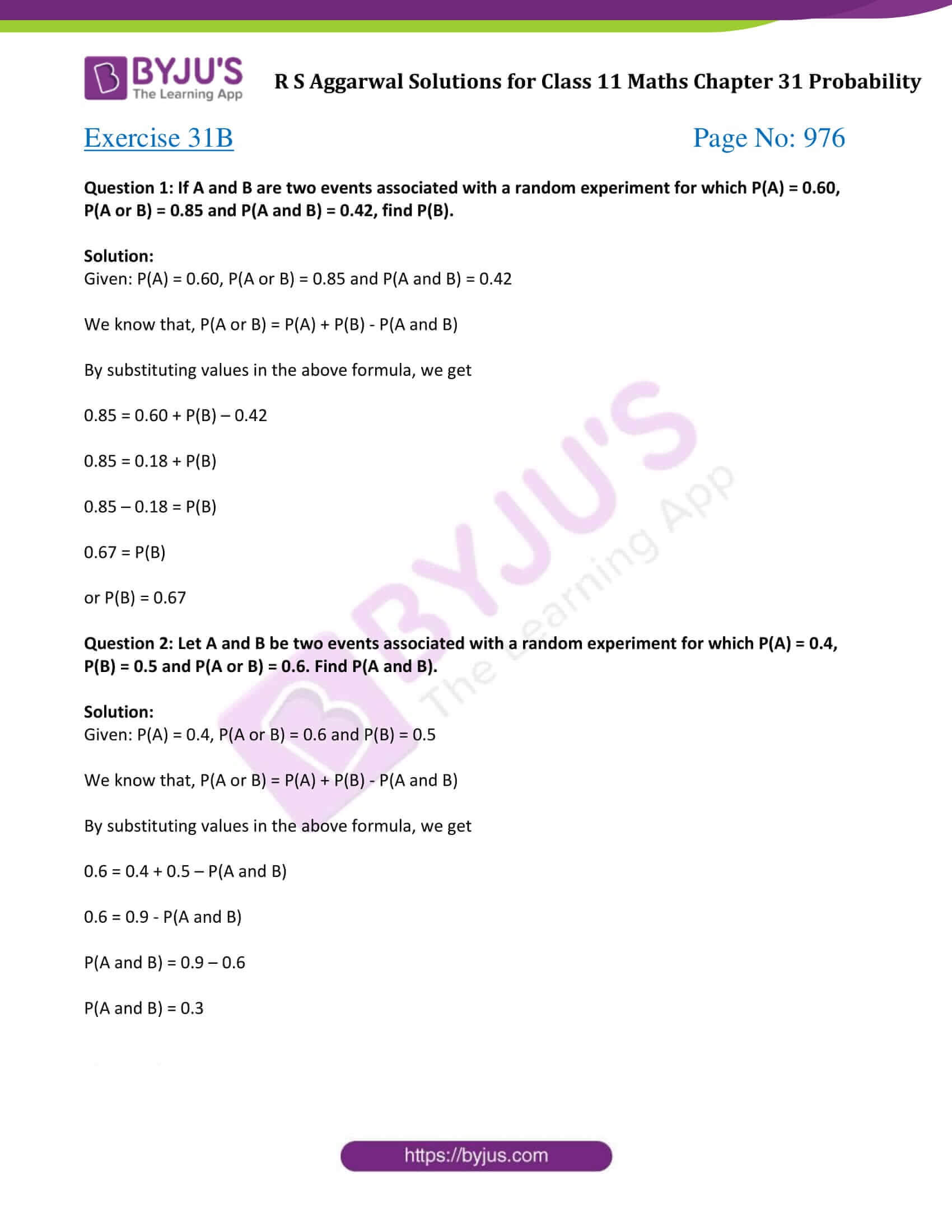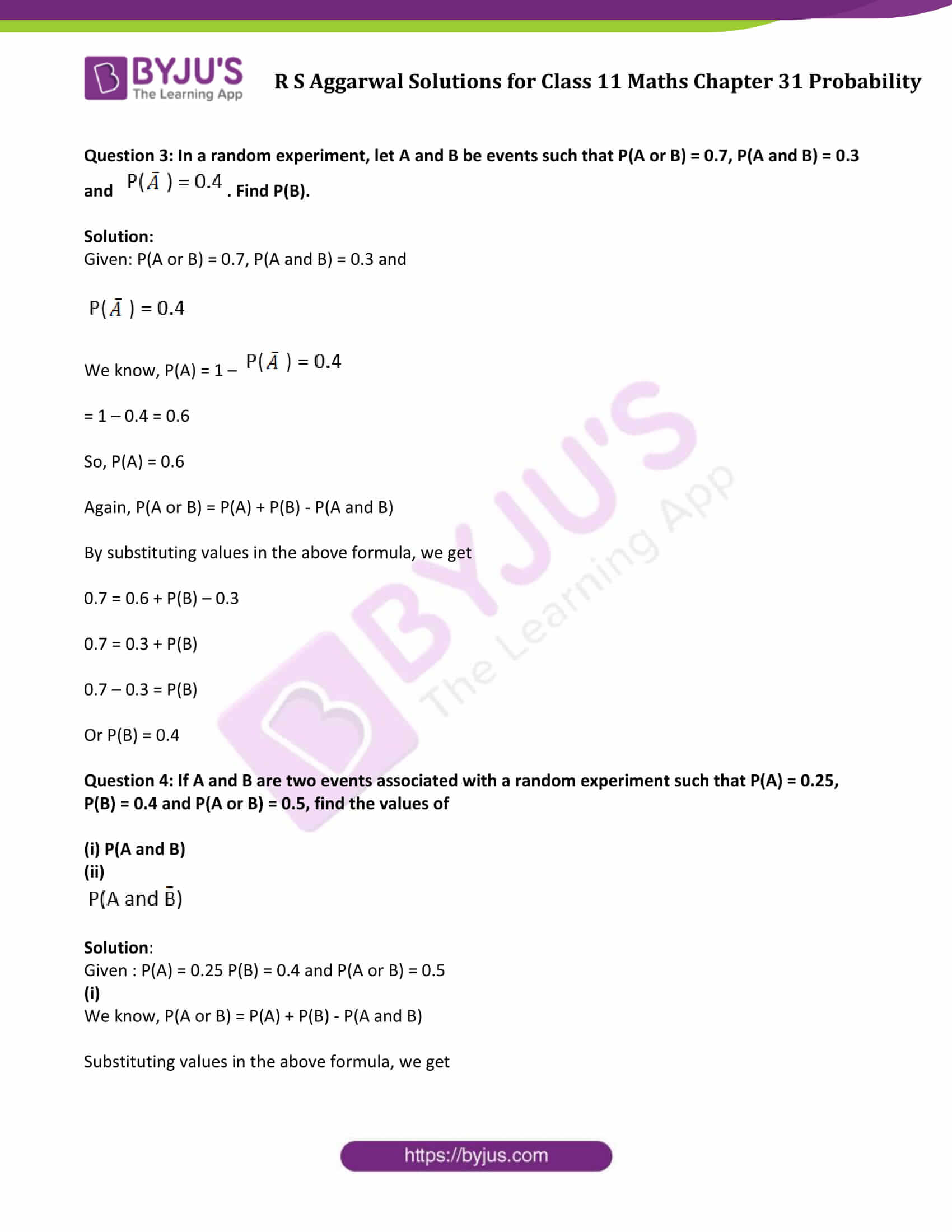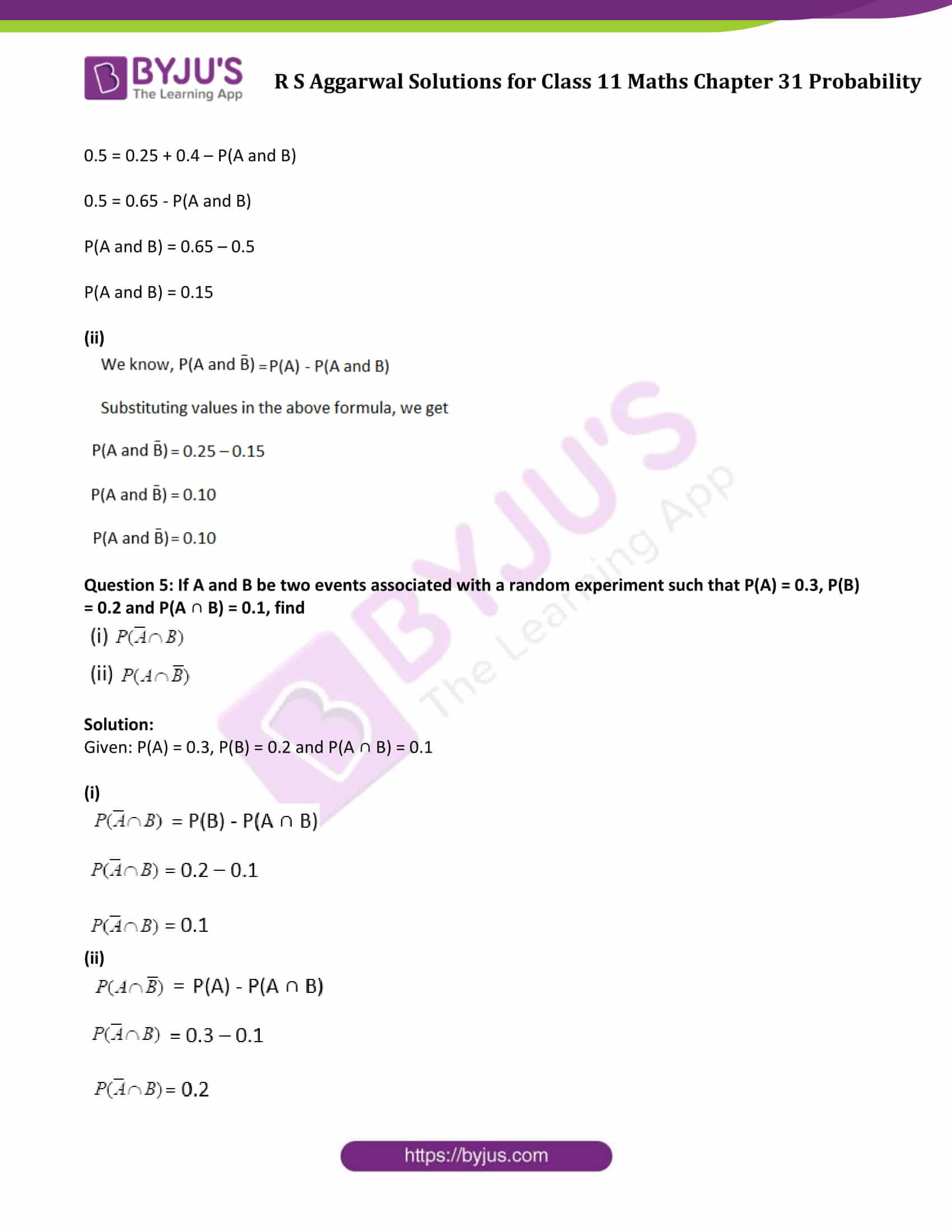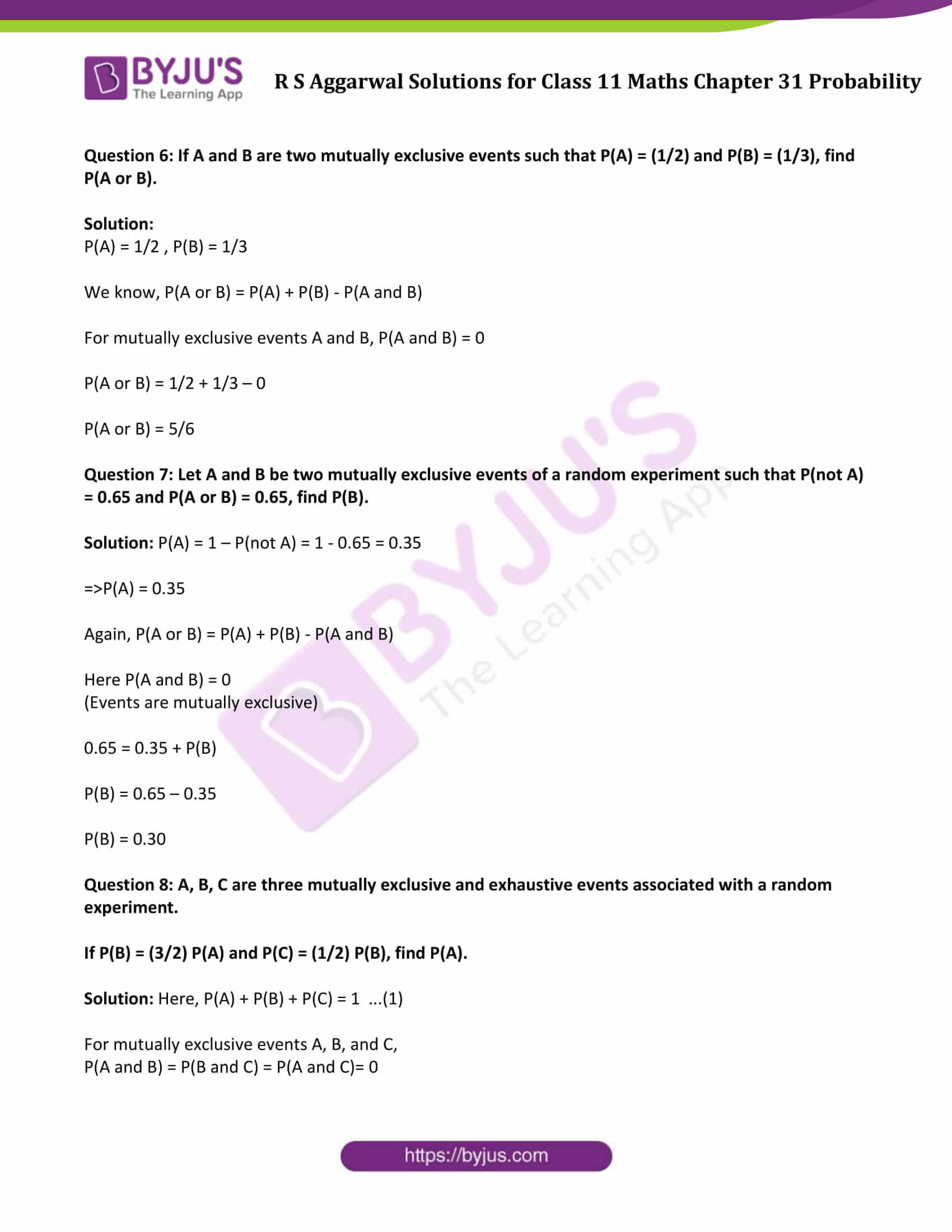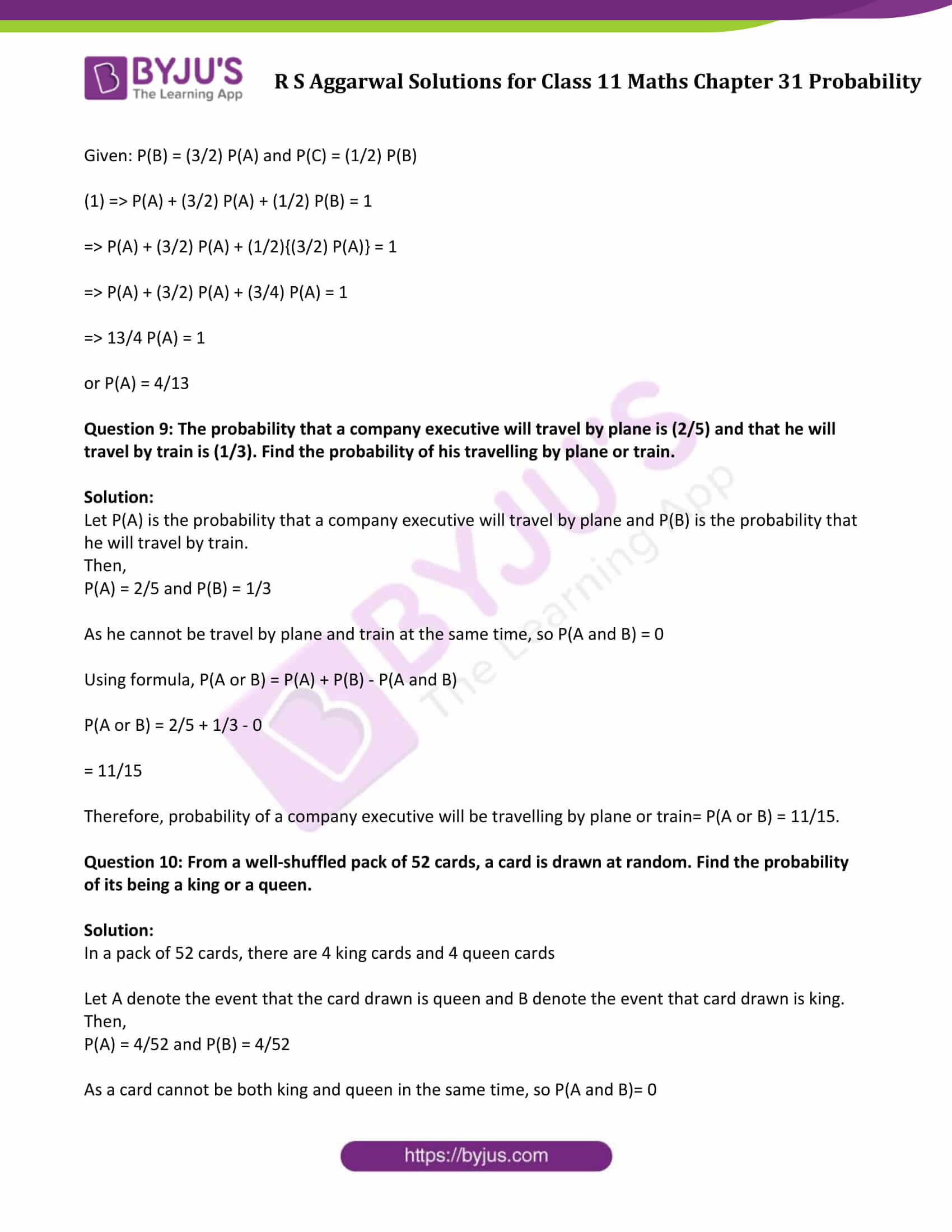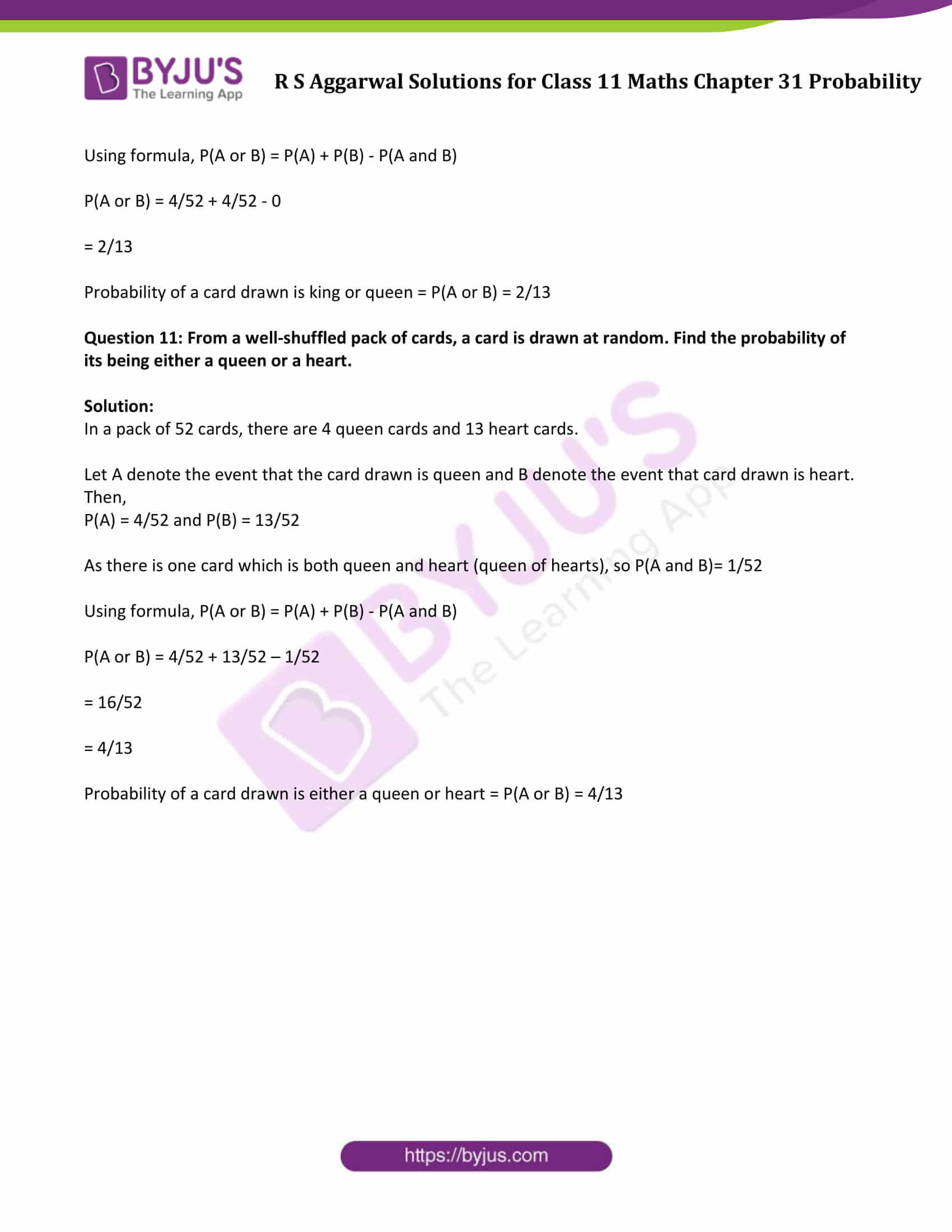### Access Answers to Maths R S Aggarwal Class 11 Chapter 31 Probability Exercise 31B Page number 976

Question 1: If A and B are two events associated with a random experiment for which P(A) = 0.60, P(A or B) = 0.85 and P(A and B) = 0.42, find P(B).

Solution:

Given: P(A) = 0.60, P(A or B) = 0.85 and P(A and B) = 0.42

We know that, P(A or B) = P(A) + P(B) – P(A and B)

By substituting values in the above formula, we get

0.85 = 0.60 + P(B) – 0.42

0.85 = 0.18 + P(B)

0.85 – 0.18 = P(B)

0.67 = P(B)

or P(B) = 0.67

Question 2: Let A and B be two events associated with a random experiment for which P(A) = 0.4, P(B) = 0.5 and P(A or B) = 0.6. Find P(A and B).

Solution:

Given: P(A) = 0.4, P(A or B) = 0.6 and P(B) = 0.5

We know that, P(A or B) = P(A) + P(B) – P(A and B)

By substituting values in the above formula, we get

0.6 = 0.4 + 0.5 – P(A and B)

0.6 = 0.9 – P(A and B)

P(A and B) = 0.9 – 0.6

P(A and B) = 0.3

Question 3: In a random experiment, let A and B be events such that P(A or B) = 0.7, P(A and B) = 0.3 and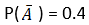. Find P(B).

Solution:

Given: P(A or B) = 0.7, P(A and B) = 0.3 andWe know, P(A) = 1 –= 1 – 0.4 = 0.6

So, P(A) = 0.6

Again, P(A or B) = P(A) + P(B) – P(A and B)

By substituting values in the above formula, we get

0.7 = 0.6 + P(B) – 0.3

0.7 = 0.3 + P(B)

0.7 – 0.3 = P(B)

Or P(B) = 0.4

Question 4: If A and B are two events associated with a random experiment such that P(A) = 0.25, P(B) = 0.4 and P(A or B) = 0.5, find the values of

(i) P(A and B)

(ii)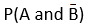Solution:

Given : P(A) = 0.25 P(B) = 0.4 and P(A or B) = 0.5

(i)

We know, P(A or B) = P(A) + P(B) – P(A and B)

Substituting values in the above formula, we get

0.5 = 0.25 + 0.4 – P(A and B)

0.5 = 0.65 – P(A and B)

P(A and B) = 0.65 – 0.5

P(A and B) = 0.15

(ii)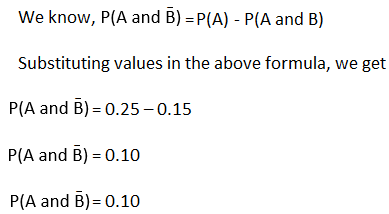Question 5: If A and B be two events associated with a random experiment such that P(A) = 0.3, P(B) = 0.2 and P(A ∩ B) = 0.1, find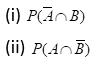Solution:

Given: P(A) = 0.3, P(B) = 0.2 and P(A ∩ B) = 0.1

(i)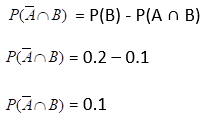(ii)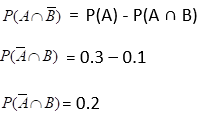Question 6: If A and B are two mutually exclusive events such that P(A) = (1/2) and P(B) = (1/3), find P(A or B).

Solution:

P(A) = 1/2 , P(B) = 1/3

We know, P(A or B) = P(A) + P(B) – P(A and B)

For mutually exclusive events A and B, P(A and B) = 0

P(A or B) = 1/2 + 1/3 – 0

P(A or B) = 5/6

Question 7: Let A and B be two mutually exclusive events of a random experiment such that P(not A) = 0.65 and P(A or B) = 0.65, find P(B).

Solution: P(A) = 1 – P(not A) = 1 – 0.65 = 0.35

=>P(A) = 0.35

Again, P(A or B) = P(A) + P(B) – P(A and B)

Here P(A and B) = 0

(Events are mutually exclusive)

0.65 = 0.35 + P(B)

P(B) = 0.65 – 0.35

P(B) = 0.30

Question 8: A, B, C are three mutually exclusive and exhaustive events associated with a random experiment.

If P(B) = (3/2) P(A) and P(C) = (1/2) P(B), find P(A).

Solution: Here, P(A) + P(B) + P(C) = 1 …(1)

For mutually exclusive events A, B, and C,

P(A and B) = P(B and C) = P(A and C)= 0

Given: P(B) = (3/2) P(A) and P(C) = (1/2) P(B)

(1) => P(A) + (3/2) P(A) + (1/2) P(B) = 1

=> P(A) + (3/2) P(A) + (1/2){(3/2) P(A)} = 1

=> P(A) + (3/2) P(A) + (3/4) P(A) = 1

=> 13/4 P(A) = 1

or P(A) = 4/13

Question 9: The probability that a company executive will travel by plane is (2/5) and that he will travel by train is (1/3). Find the probability of his travelling by plane or train.

Solution:

Let P(A) is the probability that a company executive will travel by plane and P(B) is the probability that he will travel by train.

Then,

P(A) = 2/5 and P(B) = 1/3

As he cannot be travel by plane and train at the same time, so P(A and B) = 0

Using formula, P(A or B) = P(A) + P(B) – P(A and B)

P(A or B) = 2/5 + 1/3 – 0

= 11/15

Therefore, probability of a company executive will be travelling by plane or train= P(A or B) = 11/15.

Question 10: From a well-shuffled pack of 52 cards, a card is drawn at random. Find the probability of its being a king or a queen.

Solution:

In a pack of 52 cards, there are 4 king cards and 4 queen cards

Let A denote the event that the card drawn is queen and B denote the event that card drawn is king.

Then,

P(A) = 4/52 and P(B) = 4/52

As a card cannot be both king and queen in the same time, so P(A and B)= 0

Using formula, P(A or B) = P(A) + P(B) – P(A and B)

P(A or B) = 4/52 + 4/52 – 0

= 2/13

Probability of a card drawn is king or queen = P(A or B) = 2/13

Question 11: From a well-shuffled pack of cards, a card is drawn at random. Find the probability of its being either a queen or a heart.

Solution:

In a pack of 52 cards, there are 4 queen cards and 13 heart cards.

Let A denote the event that the card drawn is queen and B denote the event that card drawn is heart.

Then,

P(A) = 4/52 and P(B) = 13/52

As there is one card which is both queen and heart (queen of hearts), so P(A and B)= 1/52

Using formula, P(A or B) = P(A) + P(B) – P(A and B)

P(A or B) = 4/52 + 13/52 – 1/52

= 16/52

= 4/13

Probability of a card drawn is either a queen or heart = P(A or B) = 4/13

## Access other exercise solutions of Class 11 Maths Chapter 31 Probability

Exercise 31A Solutions